## Exploratory Data Analysis with Pandas

This post is exploratory data analysis with pandas. Clear data plots that explicate the relationship between variables can lead to the creation of newer and better features that can predict more than the existing ones.

Exploratory Data Analysis, which can be effective if it has the following characteristics:

• It should be fast, allowing you to explore, develop new ideas and test them, and restart with a new exploration and fresh ideas.

• It should be graphic in order to better represent data as a whole, no matter how high its dimensionality is.

Instead, if your purpose is to best communicate the findings by using beautiful visualization, you may notice that it is not so easy to customize the pandas graphical outputs. Therefore, when it is paramount to create specific graphic outputs, it is better to start working directly from the beginning with matplotlib instructions.

In :
```import pandas as pd
%matplotlib inline
print  ('Your pandas version is: %s' % pd.__version__)
iris_df = pd.DataFrame(iris.data, columns=iris.feature_names)
groups = list(iris.target)
iris_df['groups'] = pd.Series([iris.target_names[k] for k in groups])
```
```Your pandas version is: 0.18.0
```
Boxplots and histograms
Distributions should always be the first aspect to be checked in your data. Boxplots draft the key figures in the distribution and help you spot outliers.
In :
```boxplots = iris_df.boxplot(return_type='axes')
```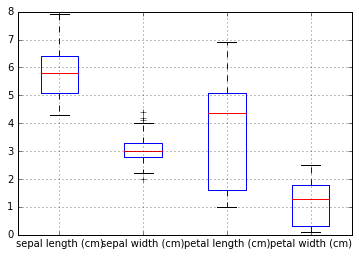If you already have groups in your data (from categorical variables, or they may be derived from unsupervised learning), just point out the variable for which you need the boxplot and specify that you need to have the data separated by the groups (use the by parameter followed by the string name of the grouping variable):

In :
```boxplots = iris_df.boxplot(column='sepal length (cm)', by='groups', return_type='axes')
```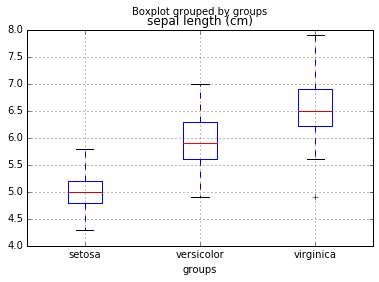In this way, you can quickly know whether the variable is a good discriminator of the group differences.

Anyway, Boxplots cannot provide you with a complete view of distributions as histograms and density plots. For instance, by using histograms and density plots, you can figure out whether there are distribution peaks or valleys.

You can obtain both histograms and density plots by using the plot method. This method allows you to represent the whole dataset, specific groups of variables (you just have to provide a list of the string names and do some fancy indexing), or even single variables.

In :
```densityplot = iris_df.plot(kind='density')
```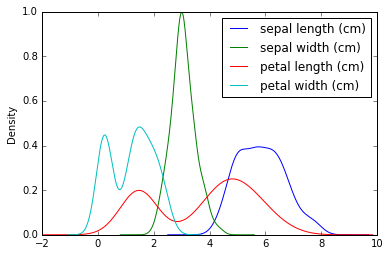In :
```single_distribution = iris_df['petal width (cm)'].plot(kind='hist', alpha=0.5)
```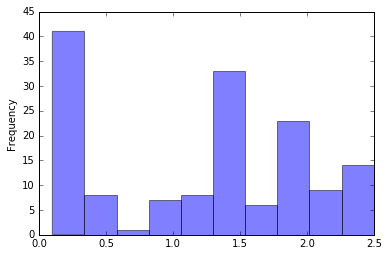## Scatterplots

Scatterplots can be used to effectively understand whether the variables are in a nonlinear relationship, and you can get an idea about their best possible transformations to achieve linearization

In :
```colors_palette = {0: 'red', 1: 'yellow', 2:'blue'}
colors = [colors_palette[c] for c in groups]
simple_scatterplot = iris_df.plot(kind='scatter', x=0, y=1, c=colors)
```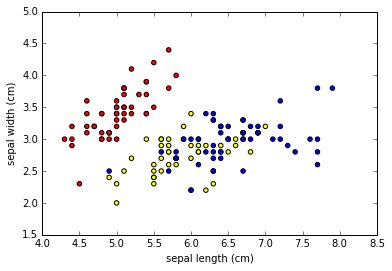Scatterplots can be turned into hexagonal binning plots. Also, they help you effectively visualize the point densities, thus revealing natural clusters hidden in your data by using some of the variables in the dataset or the dimensions obtained by PCA or other dimensionality reduction algorithm

In :
```hexbin = iris_df.plot(kind='hexbin', x=0, y=1, gridsize=10)
```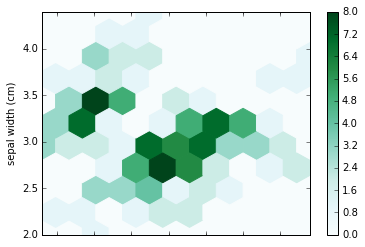The scatterplot matrix can inform you about the conjoint distributions of your features. It thus helps you locate groups in data and verify their separability.

If your variables are less in number (otherwise, the visualization will get cluttered), a quick turnaround is to automatically place a command to draw a matrix of scatterplots:

In :
```from pandas.tools.plotting import scatter_matrix
colors_palette = {0: "red", 1: "green", 2: "blue"}
colors = [colors_palette[c] for c in groups]
matrix_of_scatterplots = scatter_matrix(iris_df, alpha=0.2, figsize=(6, 6), color=colors, diagonal='kde')
```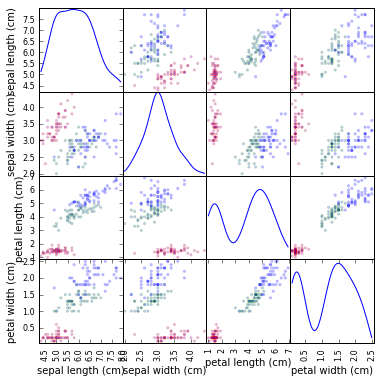The alpha parameter controls the amount of transparency, and figsize provides the width and height of the matrix in inches. Finally, color accepts a list indicating the color of each point in the plot, thus allowing the depicting of different groups in data. Also, by selecting ‘kde’ or ‘hist’ on your diagonal parameter, you can opt to represent density curves or histograms (faster) of each variable on the diagonal of the scatter matrix.

Parallel coordinates
Parallel coordinates plot is helpful in the task of providing you with a hint about the most group-discriminating variables.

By plotting all the observations as parallel lines with respect to all the possible variables (arbitrarily aligned on the abscissa), parallel coordinates will help you spot whether there are streams of observations grouped as your classes and understand the variables that best separate the streams (the most useful predictor variables).

The parallel_coordinates is a pandas function and, to work properly, it just needs as parameters the data DataFrame and the string name of the variable containing the groups whose separability you want to test. This is why you should add it to your dataset. However, don’t forget to remove it after you finish exploring by using the DataFrame.drop(‘variable name’,axis=1) method.

In :
```from pandas.tools.plotting import parallel_coordinates
iris_df['groups'] = [iris.target_names[k] for k in groups]
pll = parallel_coordinates(iris_df,'groups')
```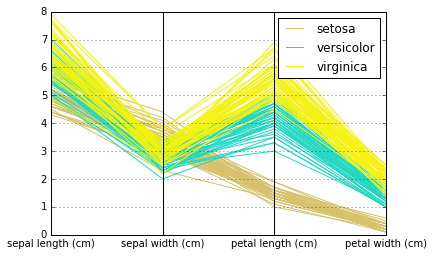This site uses Akismet to reduce spam. Learn how your comment data is processed.